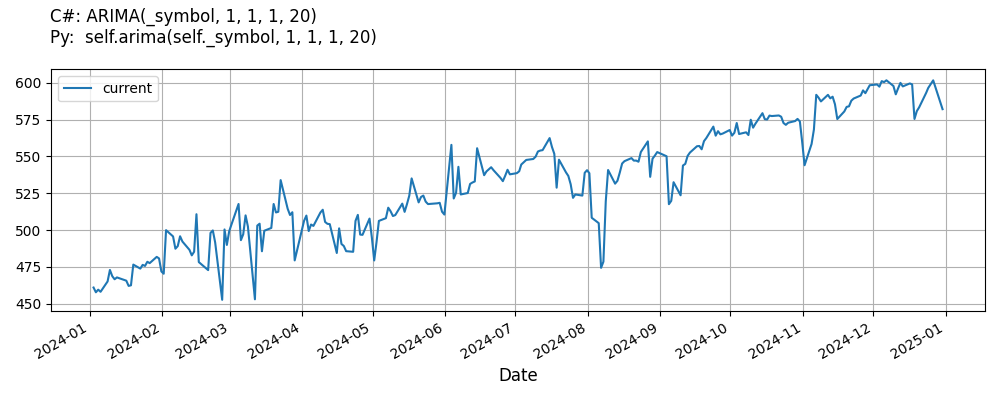# Supported Indicators

## Auto Regressive Integrated Moving Average

### Introduction

An Autoregressive Intergrated Moving Average (ARIMA) is a time series model which can be used to describe a set of data. In particular,with Xₜ representing the series, the model assumes the data are of form (after differencing times): Xₜ = c + εₜ + ΣᵢφᵢXₜ₋ᵢ + Σᵢθᵢεₜ₋ᵢ where the first sum has an upper limit of and the second .

To view the implementation of this indicator, see the LEAN GitHub repository.

### Using ARIMA Indicator

To create an automatic indicators for AutoRegressiveIntegratedMovingAverage, call the ARIMA helper method from the QCAlgorithm class. The ARIMA method creates a AutoRegressiveIntegratedMovingAverage object, hooks it up for automatic updates, and returns it so you can used it in your algorithm. In most cases, you should call the helper method in the Initialize method.

public class AutoRegressiveIntegratedMovingAverageAlgorithm : QCAlgorithm
{
private Symbol _symbol;
private AutoRegressiveIntegratedMovingAverage _arima;

public override void Initialize()
{
_arima = ARIMA(_symbol, 1, 1, 1, 20);
}

public override void OnData(Slice data)
{
{
// The current value of _arima is represented by itself (_arima)
// or _arima.Current.Value
Plot("AutoRegressiveIntegratedMovingAverage", "arima", _arima);
// Plot all properties of arima
Plot("AutoRegressiveIntegratedMovingAverage", "arresidualerror", _arima.ArResidualError);
Plot("AutoRegressiveIntegratedMovingAverage", "maresidualerror", _arima.MaResidualError);
}
}
}
class AutoRegressiveIntegratedMovingAverageAlgorithm(QCAlgorithm):
def Initialize(self) -> None:
self.arima = self.ARIMA(self.symbol, 1, 1, 1, 20)

def OnData(self, slice: Slice) -> None:
# The current value of self.arima is represented by self.arima.Current.Value
self.Plot("AutoRegressiveIntegratedMovingAverage", "arima", self.arima.Current.Value)
# Plot all attributes of self.arima
self.Plot("AutoRegressiveIntegratedMovingAverage", "arresidualerror", self.arima.ArResidualError)
self.Plot("AutoRegressiveIntegratedMovingAverage", "maresidualerror", self.arima.MaResidualError)


The following reference table describes the ARIMA method:

### ARIMA()1/1

            AutoRegressiveIntegratedMovingAverage QuantConnect.Algorithm.QCAlgorithm.ARIMA (
Symbol                           symbol,
Int32                            arOrder,
Int32                            diffOrder,
Int32                            maOrder,
Int32                            period,
*Nullable<Resolution>      resolution,
*Func<IBaseData, Decimal>  selector
)


Creates a new ARIMA indicator.

If you don't provide a resolution, it defaults to the security resolution. If you provide a resolution, it must be greater than or equal to the resolution of the security. For instance, if you subscribe to hourly data for a security, you should update its indicator with data that spans 1 hour or longer.

You can manually create a AutoRegressiveIntegratedMovingAverage indicator, so it doesn't automatically update. Manual indicators let you update their values with any data you choose.

Updating your indicator manually enables you to control when the indicator is updated and what data you use to update it. To manually update the indicator, call the Update method with time/number pair, or an IndicatorDataPoint. The indicator will only be ready after you prime it with enough data.

public class AutoRegressiveIntegratedMovingAverageAlgorithm : QCAlgorithm
{
private Symbol _symbol;
private AutoRegressiveIntegratedMovingAverage _arima;

public override void Initialize()
{
_arima = new AutoRegressiveIntegratedMovingAverage(1, 1, 1, 20, True);
}

public override void OnData(Slice data)
{
if (data.Bars.TryGeValue(_symbol, out var bar))
{
_arima.Update(bar.EndTime, bar.Close);
}

{
// The current value of _arima is represented by itself (_arima)
// or _arima.Current.Value
Plot("AutoRegressiveIntegratedMovingAverage", "arima", _arima);
// Plot all properties of arima
Plot("AutoRegressiveIntegratedMovingAverage", "arresidualerror", _arima.ArResidualError);
Plot("AutoRegressiveIntegratedMovingAverage", "maresidualerror", _arima.MaResidualError);
}
}
}
class AutoRegressiveIntegratedMovingAverageAlgorithm(QCAlgorithm):
def Initialize(self) -> None:
self.arima = AutoRegressiveIntegratedMovingAverage(1, 1, 1, 20, True)

def OnData(self, slice: Slice) -> None:
bar = slice.Bars.get(self.symbol)
if bar:
self.arima.Update(bar.EndTime, bar.Close)

# The current value of self.arima is represented by self.arima.Current.Value
self.Plot("AutoRegressiveIntegratedMovingAverage", "arima", self.arima.Current.Value)
# Plot all attributes of self.arima
self.Plot("AutoRegressiveIntegratedMovingAverage", "arresidualerror", self.arima.ArResidualError)
self.Plot("AutoRegressiveIntegratedMovingAverage", "maresidualerror", self.arima.MaResidualError)


To register a manual indicator for automatic updates with the security data, call the RegisterIndicator method.

public class AutoRegressiveIntegratedMovingAverageAlgorithm : QCAlgorithm
{
private Symbol _symbol;
private AutoRegressiveIntegratedMovingAverage _arima;

public override void Initialize()
{
_arima = new AutoRegressiveIntegratedMovingAverage(1, 1, 1, 20, True);
RegisterIndicator(_symbol, _arima, Resolution.Daily);
}

public override void OnData(Slice data)
{
{
// The current value of _arima is represented by itself (_arima)
// or _arima.Current.Value
Plot("AutoRegressiveIntegratedMovingAverage", "arima", _arima);

}
}
}
class AutoRegressiveIntegratedMovingAverageAlgorithm(QCAlgorithm):
def Initialize(self) -> None:
self.arima = AutoRegressiveIntegratedMovingAverage(1, 1, 1, 20, True)
self.RegisterIndicator(self.symbol, self.arima, Resolution.Daily)

def OnData(self, slice: Slice) -> None:
# The current value of self.arima is represented by self.arima.Current.Value
self.Plot("AutoRegressiveIntegratedMovingAverage", "arima", self.arima.Current.Value)



The following reference table describes the AutoRegressiveIntegratedMovingAverage constructor:

### AutoRegressiveIntegratedMovingAverage()1/2

            AutoRegressiveIntegratedMovingAverage QuantConnect.Indicators.AutoRegressiveIntegratedMovingAverage (
string  name,
int     arOrder,
int     diffOrder,
int     maOrder,
int     period,
*bool   intercept
)


This particular constructor fits the model by means of TwoStepFit for a specified name.

### AutoRegressiveIntegratedMovingAverage()2/2

            AutoRegressiveIntegratedMovingAverage QuantConnect.Indicators.AutoRegressiveIntegratedMovingAverage (
int   arOrder,
int   diffOrder,
int   maOrder,
int   period,
bool  intercept
)


This particular constructor fits the model by means of TwoStepFit using ordinary least squares.

### Visualization

The following image shows plot values of selected properties of AutoRegressiveIntegratedMovingAverage using the plotly library.You can also see our Videos. You can also get in touch with us via Discord.